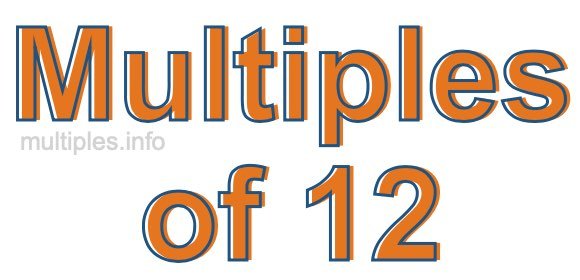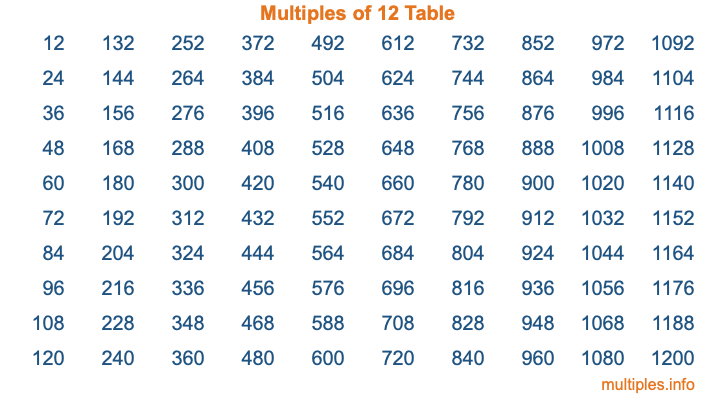Multiples of 12Welcome to the Multiples of 12 page. Here we will first teach you everything you will ever need to know about the multiples of 12, and then give you a study guide summary of everything we taught you to make sure you remember it all. Use this page to look up facts and learn information about the multiples of 12. This page will make you a multiples of twelve expert!

Definition of Multiples of 12
Multiples of 12 are all the numbers that when divided by 12 equal an integer. Each of the multiples of 12 are called a multiple. A multiple of 12 is created by multiplying 12 by an integer.

Therefore, to create a list of multiples of 12, you start with 1 multiplied by 12, then 2 multiplied by 12, then 3 multiplied by 12, and so on for as long as you want. Thus, the list of the first five multiples of 12 is 12, 24, 36, 48, and 60. To see a larger list of multiples of 12, see the printable image of Multiples of 12 further down on this page. We also have a category where you can choose any nth multiple of 12.

Multiples of 12 Checker
The Multiples of 12 Checker below checks to see if any number of your choice is a multiple of 12. In other words, it checks to see if there is any number (integer) that when multiplied by 12 will equal your number. To do that, we divide your number by 12. If the the quotient is an integer, then your number is a multiple of 12.

Is  a multiple of 12?

Least Common Multiple of 12 and ...
A Least Common Multiple (LCM) is the lowest multiple that two or more numbers have in common. This is also called the smallest common multiple or lowest common multiple and is useful to know when you are adding our subtracting fractions. Enter one or more numbers below (12 is already entered) to find the LCM.

Check out our LCM Calculator if you need more details about the Least Common Multiple or if you need the LCM for different numbers for adding and subtraction fractions.

nth Multiple of 12
As we stated above, 12 is the first multiple of 12, 24 is the second multiple of 12, 36 is the third multiple of 12, and so on. Enter a number below to find the nth multiple of 12.

th multiple of 12

Multiples of 12 vs Factors of 12
12 is a multiple of 12 and a factor of 12, but that is where the similarities end. All postive multiples of 12 are 12 or greater than 12. All positive factors of 12 are 12 or less than 12.

Below is the beginning list of multiples of 12 and the factors of 12 so you can compare:

Multiples of 12: 12, 24, 36, 48, 60, etc.

Factors of 12: 1, 2, 3, 4, 6, 12

As you can see, the multiples of 12 are all the numbers that you can divide by 12 to get a whole number. The factors of 12, on the other hand, are all the whole numbers that you can multiply by another whole number to get 12.

It's also interesting to note that if a number (x) is a factor of 12, then 12 will also be a multiple of that number (x).

Multiples of 12 vs Divisors of 12
The divisors of 12 are all the integers that 12 can be divided by evenly. Below is a list of the divisors of 12.

Divisors of 12: 1, 2, 3, 4, 6, 12

The interesting thing to note here is that if you take any multiple of 12 and divide it by a divisor of 12, you will see that the quotient is an integer.

Multiples of 12 Table
Below is an image of the first 100 multiples of 12 in a table. The table is in chronological order, column by column. The first column has the first ten multiples of 12, the second column has the next ten multiples of 12, and so on.The Multiples of 12 Table is also referred to as the 12 Times Table or Times Table of 12. You are welcome to print out our table for your studies.

Negative Multiples of 12
Although not often discussed or needed in math, it is worth mentioning that you can make a list of negative multiples of 12 by multiplying 12 by -1, then by -2, then by -3, and so on, to get the following list of negative multiples of 12:

-12, -24, -36, -48, -60, etc.

Multiples of 12 Summary
Below is a summary of important Multiples of 12 facts that we have discussed on this page. To retain the knowledge on this page, we recommend that you read through the summary and explain to yourself or a study partner why they hold true.

There are an infinite number of multiples of 12.

A multiple of 12 divided by 12 will equal a whole number.

12 divided by a factor of 12 equals a divisor of 12.

The nth multiple of 12 is n times 12.

The largest factor of 12 is equal to the first positive multiple of 12.

12 is a multiple of every factor of 12.

12 is a multiple of 12.

A multiple of 12 divided by a divisor of 12 equals an integer.

12 divided by a divisor of 12 equals a factor of 12.

Any integer times 12 will equal a multiple of 12.

Multiples of a Number
Here you can get the multiples of another number, all with the same attention to detail as we did for multiples of 12 on this page.

Multiples of
Multiples of 13
Did you find our page about multiples of twelve educational? Do you want more knowledge? Check out the multiples of the next number on our list!